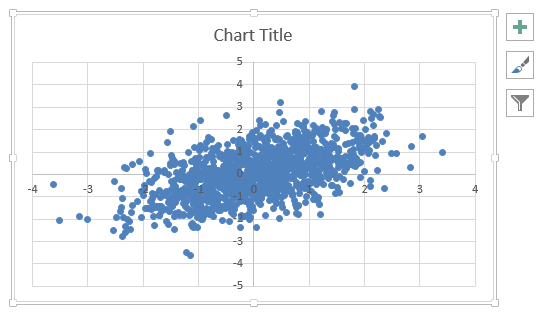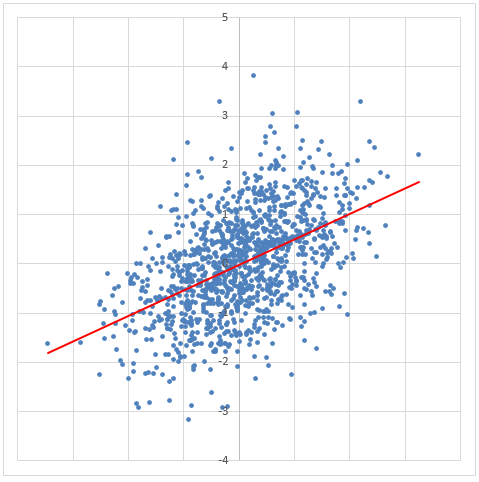# x-y scatter plot with correlated random numbers

In this module:

• Random number generator – using volatile functions – with number of observations $$n$$ switch
• Dynamic range as a statistical data reference
• Dynamic range as a chart series
• Creating an X Y scatter chart

## Random number generator

Pseudo random numbers $$\text{N(0,1) i.i.d.}$$ are generated by the Excel RAND function and then passed as the probability argument to the NORM.S.INV function. The correlation structure is incorporated by using the Cholesky decomposition method.

### Cholesky decomposition

Generate $$Z_1,Z_2$$

1. Generate $$Z_1,Z_2 \sim \text{N(0,1) i.i.d.}$$
2. Set $$X=Z_1$$ and $$Y=\rho Z_1 + Z_2 \sqrt{1-\rho^2}$$, where $$\rho$$ is the correlation coefficient

### The X Y data worksheet

$$Z_1,Z_2$$ are generated on the worksheet named: X Y data. Pseudo random numbers $$\text{N(0,1) i.i.d.}$$ are generated with the nested NORM.S.INV(RAND()) formula.

1. Cell B3: =IF(ROW()-2<=NoObs,NORM.S.INV(RAND()),"")
2. Cell C3: =IF(ROW()-2<=NoObs,rho*B3+SQRT(1-rho^2)*NORM.S.INV(RAND()),"") where rho and NoObs are links to the selector and validator panel
3. Copy B3:C3 and paste to the range B4:B5002. Name B3:B5002 as X, and C3:C5002 as Y

## Summary statistics and the X-Y scatter plot

Refer to the X Y Interface worksheet shown in the figure 1 Excel Web App #1.

### The selector / validator panel

The correlation selector

1. D5: the correlation selector - is set up by Data Validation
2. Select Data > Data Tools > Data Validation
3. In the Data Validation dialog box - Settings tab
4. Set Validation criteria; Allow: Decimal; Data: between; Minimum: -1; Maximum 1
5. In the Data Validation dialog box - Input Message tab
6. Set Title: Correlation value; Input message: Enter rho in the range ... -1 <= rho <= +1

The "No of points" validator

1. D6: the "No of point"s validator - is set up by Data Validation
2. Select Data > Data Tools > Data Validation
3. In the Data Validation dialog box - Setting tab
4. Set Validation Criteria; Allow: List; Source: 625,1250,2500,5000
5. In the Data Validation dialog box - Input Message tab
6. Set Title: Number of observations; Input message: 625, 1250, 2500, 5000, ... Select 625 to reduce worksheet recalculation time

### The descriptive statistics panel

Note: INDIRECT(D$10) uses the value in cell D10, X, to point to the range named X. Formulas in the summary statistics section: • C11: =MIN(INDIRECT(D$10)
• C12: =MAX(INDIRECT(D$10) • C13: =AVERAGE(INDIRECT(D$10)
• C14: =VAR.S(INDIRECT(D$10) • C15: =STDEV.S(INDIRECT(D$10)
• C16: =SKEW(INDIRECT(D$10) • C17: =KURT(INDIRECT(D$10)
• C18: =COUNT(INDIRECT(D$10) • C19: =MAX(INDIRECT(D$10)-MIN(INDIRECT(D$10) • C21: =COVARIANCE.S(X,Y) • C22: =CORREL(X,Y) • C23: =COVARIANCE.S(X,Y)/(STDEV.S(X)*STDEV.S(Y)) ### The X Y scatter plot 1. To select the data, type XV,YV in the Name Box. If you are using the Web App file, you will need to Unhide the X Y data worksheet before you select the data. Create the chart on that worksheet, then move it to the X Y interface worksheet 2. Insert the chart - follow the ribbon sequence: Insert > Charts > Charts Dialog Launcher > All Charts tab > X Y (Scatter) > Scatter. Click OK to display the chart shown in figure 2 3.Fig 2: X Y Scatter plot - with Number of points set to 1,250 4. Using the Insert > Chart sequence does not retain the reference to the dynamic range names, instead a static name is applied (see figure 4). When a vector length shorter than the static range is selected, the X Y chart collapses as shown in figure 3. The x axis (the Horizontal (Value) Axis) displays the observation numbers, with the observation 626 onwards displaying as blank (the static vector being 1,250 data points in length) 5.Fig 3: X Y Scatter plot - with Number of points set to 625. The scatter plot has collapsed because the Series references are static 6. To display the Edit Series dialog box, use the ribbon sequence Design > Data > Select Data to display the Select Data Source dialog box, then, in Legend Entries (Series) select Series1, then click Edit. The Edit Series dialog box will now be displayed - figure 4 7.Fig 4: X Y Scatter - Edit Series dialog box - showing that Series Values are static - hard coded references 8. In step 1, the dynamic ranges XY and YV were selected, but these were overwritten by Series X values: ='X Y data!$B$3:$B$1252 and Series Y values: ='X Y data!$C$3:$C\$1252 as shown in the Edit Series dialog box in figure 4. The series reference need to be replaced with links to the dynamic vectors
9. Link the chart series to the dynamic range - by editing the series values. Set Series X values: ='xlf-x-y-scatter.xlsx'!XV, and Series Y values: ='xlf-x-y-scatter.xlsx'!YV
10. Format the X Y chart - from the Chart Tools tab, select Format > Current Selection > Chart Elements box then the Chart Area element.
11. From the same ribbon tab, select Size, and set Height: 12.5 cm, and Width: 12.5 cm
12. A two vector X Y plot has only one series, and the Series name value in figure 4 was left blank, thus the default name is Series1. Select Series1 from the Chart Element box
13. Click Format Selection to display the Format Data Series task pane. This is the Office 2013 implementation of an Office 2010 dialog box. An example is shown in figure 5
15. Select Marker > Marker Options, then Built-in, and set Size: 2
16. Select Series Options > Horizontal (Value) Axis. This will display the Format Axis task pane
17. Select Axis Options (the three column icon), then set Bounds; Minimum: -4.0, and Maximum: 4.0. Vertical axis crosses; Axis value: 0.0
18. Repeat step 10 for the Vertical (value) Axis. Select the axis from the Axis Options > Elements List box - figure 6
20. Add a trendline - Design > Chart Layouts > Add Chart Element > Trendline > More Trendline options to display the Format Trendline task pane.
21. Select the Fill & Line tab, then set Line: Solid; Color: Standard Colors, Red; Width: 1.5 pt; Dash type: Solid; then Close the task pane.
22. Hide the chart title - Chart Tools > Design > Add Chart Elements > Chart Title > None
23. Cut and paste the chart to the X Y interface worksheet
24. The completed chart appears in figure 7
25.Fig 7: X Y scatter - completed. Includes gridlines. Selector / validator options set to correlation 0.5 and 1250 points

Selected Excel functions used in this module and associated statistical functions.

Excel functions Description
NORM.S.INV(probability)

Returns the inverse of the standard normal cumulative distribution $$\text{N(0,1) i.i.d.}$$.
RAND() Returns an evenly distributed random number $$0 \le x \lt 1$$. The rand function takes no arguments
ROW([reference]) Returns the row number of a reference
SQRT(number) Returns the positive square root of a number $$\sqrt{x}; \quad x^{0.5}$$

• This example was developed in Excel 2013 Pro 64 bit.